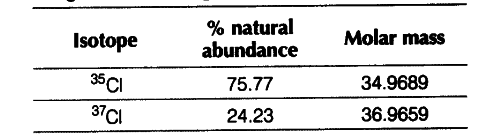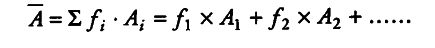# Calculate the atomic mass (average) of chlorine using the following data

Calculate the atomic mass (average) of chlorine using the following data.Average atomic mass is the sum of the products of fractional abundances of the isotopes and their corresponding mass number.
Average atomic mass,Average atomic mass,

\bar { A } = 0.7577 x 34.9689 + 0.2423 x 36.9659 = 26.4959 + 8.9568 = 35.4527